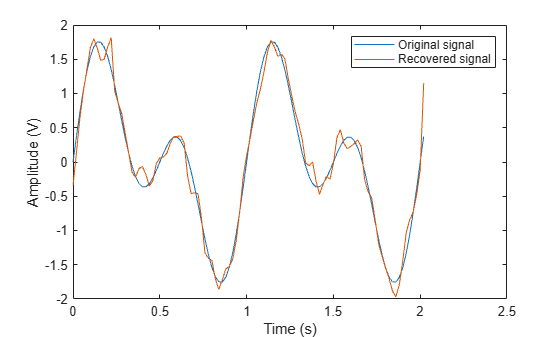# pmmod

Phase modulation

## Syntax

``y = pmmod(x,Fc,Fs,phasedev)``
``y = pmmod(x,Fc,Fs,phasedev,ini_phase)``

## Description

example

````y = pmmod(x,Fc,Fs,phasedev)` modulates the message signal `x` using phase modulation.```
````y = pmmod(x,Fc,Fs,phasedev,ini_phase)` specifies the initial phase of the modulated signal in radians.```

## Examples

collapse all

Set the sample rate. To plot the signals, create a time vector.

```fs = 50; t = (0:2*fs+1)'/fs;```

Create a sinusoidal input signal.

`x = sin(2*pi*t) + sin(4*pi*t);`

Set the carrier frequency and phase deviation.

```fc = 10; phasedev = pi/2;```

Modulate the input signal.

`tx = pmmod(x,fc,fs,phasedev);`

Pass the signal through an AWGN channel.

`rx = awgn(tx,10,'measured');`

Demodulate the noisy signal.

`y = pmdemod(rx,fc,fs,phasedev);`

Plot the original and recovered signals.

```figure; plot(t,[x y]); legend('Original signal','Recovered signal'); xlabel('Time (s)') ylabel('Amplitude (V)')```## Input Arguments

collapse all

Input signal, specified as a vector or matrix of positive integers. If `x` is a matrix, `pmmod` processes the columns independently.

Example: `sin(2*pi*t) + sin(6*pi*t)`

Data Types: `double`

Carrier frequency, specified as a positive scalar.

Data Types: `double`

Sample rate, specified as a positive scalar. `Fs` must be at least 2*`Fc`.

Data Types: `double`

Initial phase of the modulated signal (in radians), specified as a real scalar.

Example: `pi/4`

Data Types: `double`

Phase deviation, specified as a positive scalar in radians.

Data Types: `double`

## Output Arguments

collapse all

Complex baseband representation of a PM-modulated signal, returned as vector or matrix of complex values. The columns of `y` represent independent channels.

Data Types: `double` | `single`
Complex Number Support: Yes